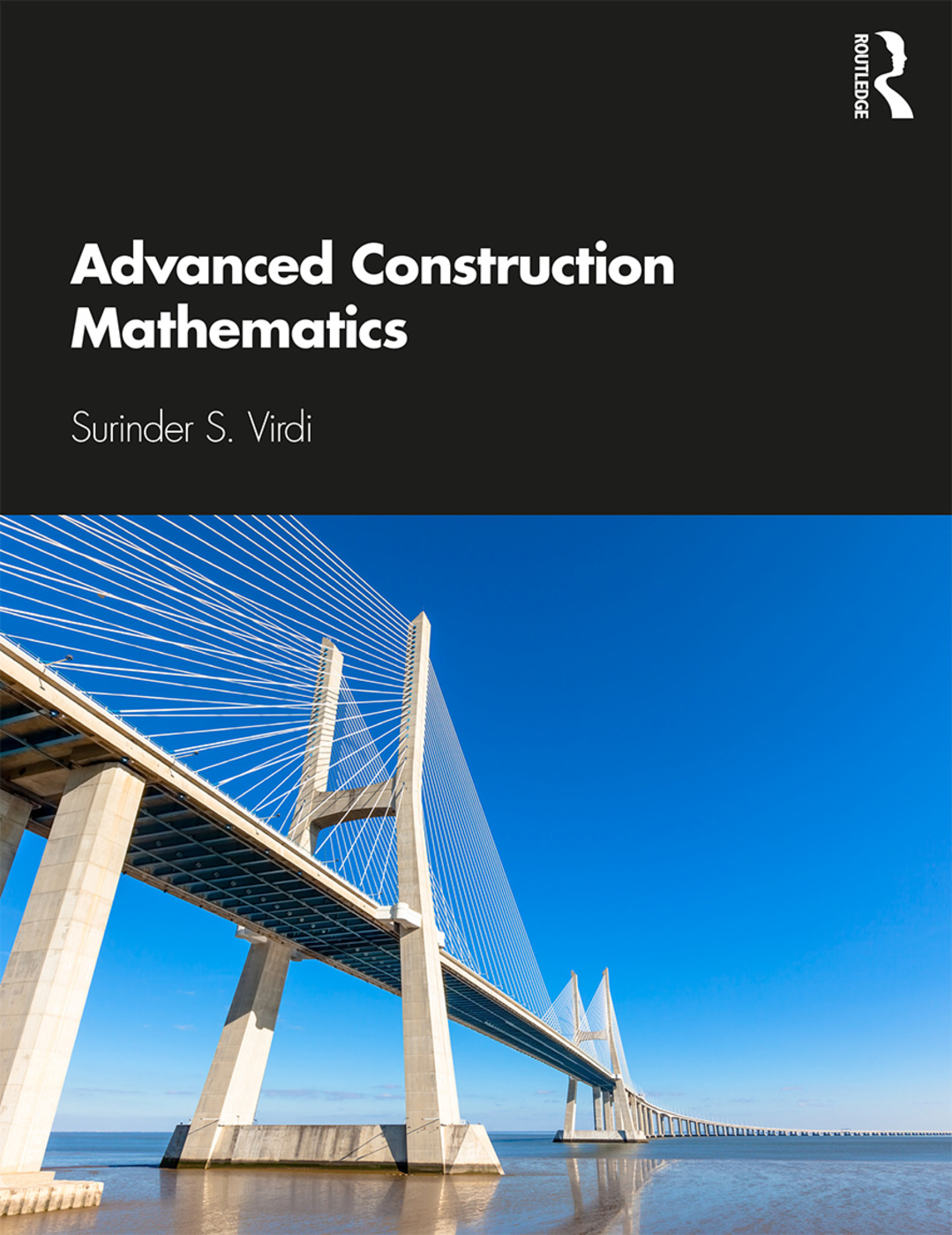1st Edition

Routledge

402 pages | 15 Color Illus. | 305 B/W Illus.

Purchasing Options:\$ = USD
Paperback: 9780367002138
pub: 2019-04-02
SAVE ~\$8.99
\$44.95
\$35.96
x
Hardback: 9780367002107
pub: 2019-04-03
SAVE ~\$27.00
\$135.00
\$108.00
x
eBook (VitalSource) : 9780429400742
pub: 2019-03-21
from \$21.48

FREE Standard Shipping!

Description

Advanced Construction Mathematics covers the range of topics that a student must learn in order to achieve success in Level 3 and 4 mathematics for the Pearson BTEC National and BTEC HNC/HND in Construction, Building Services, and Civil Engineering.

Packed with easy to follow examples, its 18 chapters cover algebra (equations, transposition and evaluation of formulae), differentiation, integration, statistics and numerous other core concepts and their application in the construction/civil engineering field. The book explains technical processes before applying mathematical techniques to solve practical problems which gradually build in complexity. Each chapter contains self-test exercises and answers and numerous illustrations to simplify the essential maths required at Levels 3 and 4. The book is also a useful recap or primer for students on BSc or non-cognate MSc Construction and Civil Engineering degrees.

Chapter 1: Introduction to basic techniques

Chapter 2: Algebra 1

Chapter 3: Algebra 2

Chapter 4: Simultaneous and Quadratic equations

Chapter 5: Graphs

Chapter 6: Geometry, Areas and Volumes

Chapter 7: Trigonometry 1

Chapter 8: Trigonometry 2

Chapter 9: Logarithmic, Exponential and Hyperbolic Functions

Chapter 10: Differentiation

Chapter 11: Applications of Differentiation

Chapter 12: Integration

Chapter 13: Applications of Integration

Chapter 14: Properties of Sections

Chapter 15: Matrices and Determinants

Chapter 16: Vectors

Chapter 17: Statistics

Chapter 18: Computer Techniques

End of Unit Assignment

Appendix 1: Bending Movement and Shear Force

Appendix 2: Frame Analysis

Appendix 3: Solutions - Exercise 1.1 to Exercise 18.1

Surinder S. Virdi is a lecturer at South and City College Birmingham, UK. He has taught construction-related topics for over 30 years and has written and co-authored two books, Construction Science and Materials, 2nd edition, 2017, and Construction Mathematics, 2nd edition, 2014.

Subject Categories

MAT000000
MATHEMATICS / General
MAT004000
MATHEMATICS / Arithmetic
MAT012000
MATHEMATICS / Geometry / General
MAT032000
MATHEMATICS / Trigonometry
TEC005000
TECHNOLOGY & ENGINEERING / Construction / General